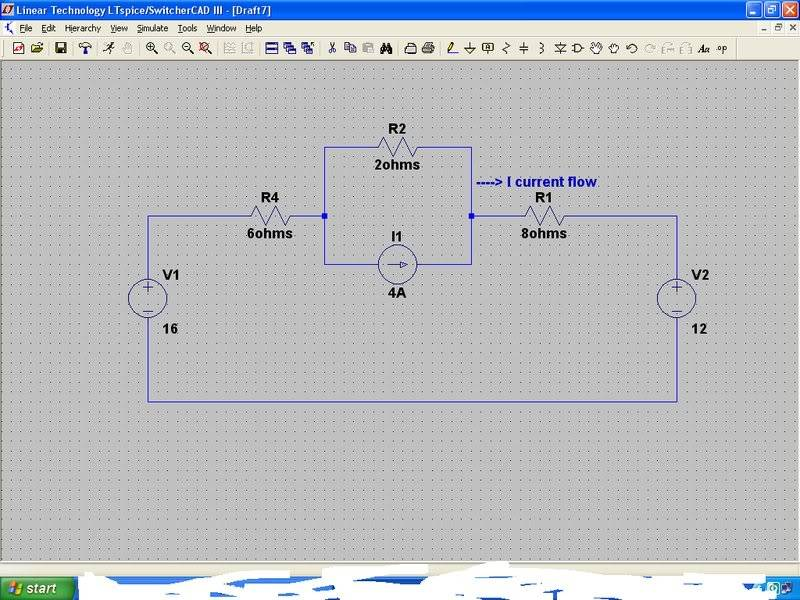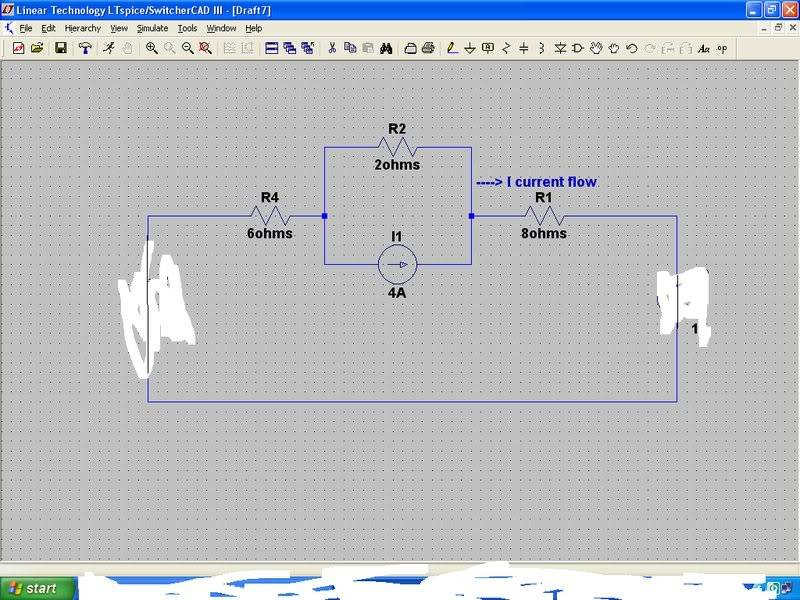# Using the superposition principle

Edwardo_Elric

## Homework Statement

Find I in the circuit using the superposition principle## Homework Equations

superposition:
I = i_1 + i_2 + i_3

## The Attempt at a Solution

for i_1 we neglect the 12V and the 4A sources:
(8 + 2 + 6)i_1 - 16 = 0
i_1 = 1A

for i_2 we neglect the 16V and the 4A sources:
(8 + 2 + 6)i_2 + 12 = 0
i_2 = -0.75

i don't actually know how to solve for the current in i_3:since there are two nodes only i use parallel resistors:
6 + 8 = 14ohms... and 2 ohms are parallel
1/14 + 1/2 = 1/R
R = 1.75
current source = 4 A
so the current source and 1.75 are in parallel... so what i need is to solve the outer current,i_3
now I am really stuck can someone help pls
ans. I = 0.75A

Last edited:

lukas86
Is there a diagram or anything? May be helpful, at least for me.

Edwardo_Elric
Is there a diagram or anything? May be helpful, at least for me.

what kind of diagram?
it is already shown: outer current of parallel(2 ohm and 4A) is required

Homework Helper
Hi Edwardo Elric,

You almost have the current for i3. You know that the 4A is splitting, with part of it going to the 2 ohm branch, and part going to the 14 ohm branch. Now the question is, if that is true, how much of the 4A goes in the 14ohm branch? That is i3.

You can find it by considering that since the ratios of the resistances are 1:7, the ratio of the currents must be 7:1. Do you get the answer?